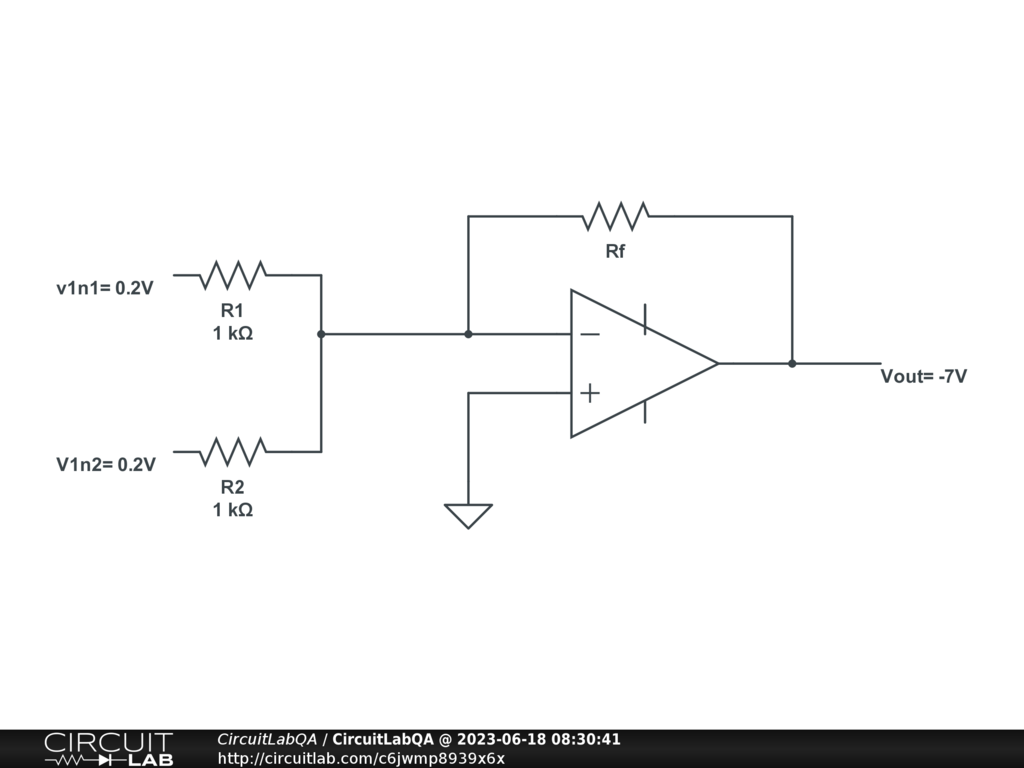## OP-AMP

 In the given OP-AMP circuit on the side: a. Find the feedback resistor (Rf) required to obtain the specified output voltage. b. If R1 = R2 = 2.2 kΩ and Rf = 18 kΩ, calculate the output voltage (VOUT).by musethor June 18, 2023 For an ideal OpAmp, the point where the inputs resistor and Rf meet all together, in this configuration, is at 0 volt (since the other input of the OpAmp is at the ground), giving the current from R1 equals to 200mV / R1, and the current from R2 is also 200mV / R2. For an ideal OpAmp, no current enters in the OpAmp through the inputs, so the incoming current coming from R1 et R2 has to exit through Rf. The current through Rf is thus 0.200/R1 + 0.200/R2 [Amp], so the voltage on the output side of Rf is Rf * (0.200/R1 + 0.200/R2) = 18000 * (0.2/2200 + 0.2/2200) = 3.27 volt. Negative. You should be able to solve the first part using a similar train of thought. by vanderghast June 18, 2023

CircuitLab's Q&A site is a FREE questions and answers forum for electronics and electrical engineering students, hobbyists, and professionals.

We encourage you to use our built-in schematic & simulation software to add more detail to your questions and answers.

Acceptable Questions:

• Concept or theory questions
• Practical engineering questions
• “Homework” questions
• Software/hardware intersection
• Best practices
• Design choices & component selection
• Troubleshooting

Unacceptable Questions:

• Non-English language content
• Non-question discussion
• Non-electronics questions
• Vendor-specific topics
• Pure software questions
• CircuitLab software support

Please respect that there are both seasoned experts and total newbies here: please be nice, be constructive, and be specific!